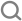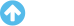A graph is plotted taking o C along the Y - axis toppr.comphysics

# A graph is plotted taking C along the Y-axis and F along the X-axis. It is a/an

A .
parabola
B .
straight line
C .
ellipse
D .
circle
December 27, 2019Muskan Larjee

0 degrees Celsius is equal to 32 degrees Fahrenheit:

0 °C = 32 °F

The temperature T in degrees Fahrenheit (°F) is equal to the temperature T in degrees Celsius (°C) times 9/5 plus 32:

T(°F) = T(°C) × 9/5 + 32

or

T(°F) = T(°C) × 1.8 + 32

A graph is plotted taking ooC along the Y-axis and ooF along the X-axis. It is a straight line415 Qs

# Absolute zero corresponds to

VIEW MORE

## Relation Between Units of Temperature

ExampleDefinitionsFormulaes
20,000+
Learning videos
8,000+
Fun stories
10,000+
Fundamental concepts
8,00,000+
Homework Questions

### Learn with VideosCalibration of Celsius, Kelvin and, Fahrenheit Scale - I
11 min

### Quick summary with StoriesStuck at a Question?